Next: Kinetic and other approximations Up: Kinetic Formulations and Regularity Previous: Velocity averaging lemmas ()

# Regularizing effect revisited (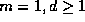)

In this section we resume our discussion on the regularization effect of nonlinear conservation laws. The averaging lemma enables us to identify the proper notion of 'nonlinearity' in the multivariate case, which guarantee compactness.

The following result, adapted from , is in the heart of matter.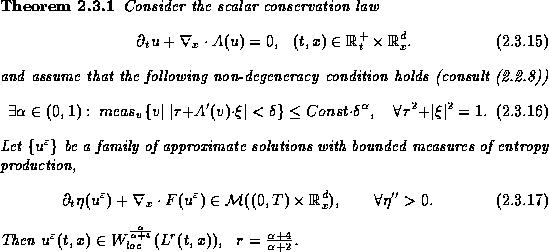Remark. Note that the bounded measure of entropy production in (2.3.17) need not be negative; general bounded measures will do.

Proof.To simplify notations, we use the customary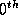index for time direction,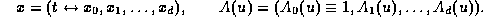The entropy condition (2.3.17) with Kruzkov entropy pairs (), reads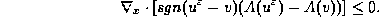This defines a family of non-negative measures,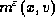,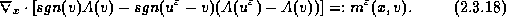Differentiate (2.3.18) w.r.t. v: one finds that the indicator function,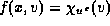, where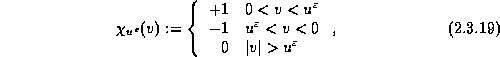satisfies the transport equation,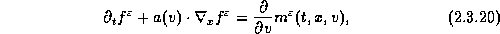which corresponds to (2.2.7) with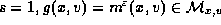. We now apply the averaging lemma with (s = q= 1, p = 2), which tells us that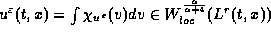as asserted.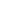It follows that if the non-degeneracy condition (2.3.16) holds, then the family of approximate solutions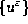is compact and strong convergence follows. In this context we refer to the convergence statement for measure-valued solutions for general multidimensional scalar conservation laws - approximate solutions measured by their nonpositive entropy production outlined in Lecture I, §.

Here, Theorem 2.3.1 yields even more, by quantifying the regularity of approximate solutions with bounded entropy productions in terms of the non-degeneracy condition (2.3.16). In fact, more can be said if the solution operator associated withis translation invariant: a bootstrap argument yields an improved regularity, ,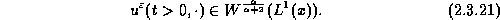In particular, if the problem is nonlinear in the sense that the non-degeneracy condition (2.2.14) holds,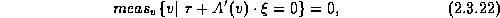then the corresponding solution operator,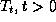, has a regularization effect mapping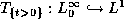. This could be viewed as a multidimensional generalization for Tartar's regularization result for a.e. nonlinear one-dimensional fluxes,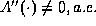.

We continue with few multidimensional examples which illustrate the relation between the non-degeneracy condition, (2.3.16) and regularity.

Example #1. The 'two-dimensional Burgers' equation' (2.1.6),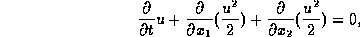has a linearized symbol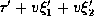which fails to satisfy the non-degeneracy/non-linearity condition (2.3.16), since it vanishes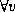's along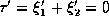. This corresponds to its persistence of oscillations along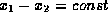, which excludes compactness.

Example #2. We consider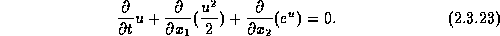In this case the linearized symbol is given by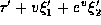; Here we have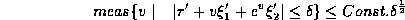(just consider the second-order touch-point at v=1). Hence, the solution operator associated with (2.3.23) is compact ( - in fact, mapping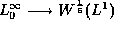.)

Example #3. Consider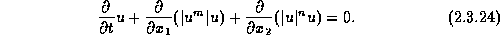For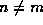we obtain an index of non-degeneracy/non-linearity of order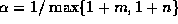.Next: Kinetic and other approximations Up: Kinetic Formulations and Regularity Previous: Velocity averaging lemmas ()